Question

# For 300 trading days, the daily closing price of a stock (in \$) is well modeled...

For 300 trading days, the daily closing price of a stock (in \$) is well modeled by a Normal model with mean \$195.61 and standard deviation \$7.15. According to this model, what cutoff value of price would separate the

a) lowest 11% of the days?

b) highest 0.86%?

c) middle 58%?

d) highest 50%?

Part a)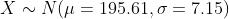P ( X < ? ) = 11% = 0.11
Looking for the probability 0.11 in standard normal table to calculate critical value Z = -1.23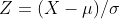-1.23 = ( X - 195.61 ) / 7.15
X = 186.82
P ( X < 186.82 ) = 0.11

Part b)P ( X > ? ) = 1 - P ( X < ? ) = 1 - 0.0086 = 0.9914
Looking for the probability 0.9914 in standard normal table to calculate critical value Z = 2.382.38 = ( X - 195.61 ) / 7.15
X = 212.63
P ( X > 212.63 ) = 0.0086

Part c)P ( a < X < b ) = 0.58
Dividing the area 0.58 in two parts we get 0.58/2 = 0.29
since 0.5 area in normal curve is above and below the mean
Area below the mean is a = 0.5 - 0.29
Area above the mean is b = 0.5 + 0.29
Looking for the probability 0.21 in standard normal table to calculate critical value Z = -0.81
Looking for the probability 0.79 in standard normal table to calculate critical value Z = 0.81-0.81 = ( X - 195.61 ) / 7.15
a = 189.82
0.81 = ( X - 195.61 ) / 7.15
b = 201.40
P ( 189.82 < X < 201.40 ) = 0.58

Part d)P ( X > ? ) = 1 - P ( X < ? ) = 1 - 0.5 = 0.5
Looking for the probability 0.5 in standard normal table to calculate critical value Z = 00 = ( X - 195.61 ) / 7.15
X = 195.61
P ( X > 195.61 ) = 0.5

#### Earn Coins

Coins can be redeemed for fabulous gifts.

Similar Homework Help Questions
• ### For 300 trading​ days, the daily closing price of a stock​ (in \$) is well modeled...

For 300 trading​ days, the daily closing price of a stock​ (in \$) is well modeled by a Normal model with mean ​\$196.12 and standard deviation ​\$7.12 According to this​model, what cutoff value of price would separate the ​a) lowest 11​% of the​ days? ​b) highest 0.77%? ​c) middle 52%? ​d) highest 50%?

• ### 7.CE.28 Question Help For 300 trading days, the daily closing price of a stock (in 5)...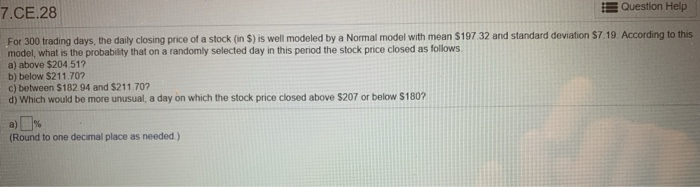7.CE.28 Question Help For 300 trading days, the daily closing price of a stock (in 5) is well modeled by a Normal model with mean S19732 and standard deviation \$7.19 According to this model, what is the probability that on a randomly selected day in this period the stock price closed as follows a) above \$204.51? b) below \$211.707 c) between \$182 94 and \$211.707 d) Which would be more unusual, a day on which the stock price closed above...

• ### The quarterly returns for a group of 52 mutual funds are well modeled by a Normal...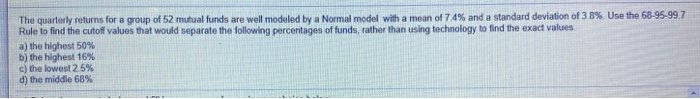The quarterly returns for a group of 52 mutual funds are well modeled by a Normal model with a mean of 74% and a standard deviation of 38% Use the 68-95-99.7 Rule to find the cutoff values that would separate the following percentages of funds, rather than using technology to find the exact values a) the highest 50% b) the highest 16% c) the lowest 25% d) the middle 68%

• ### The quarterly returns for a group of 66 mutual funds are well modeled by a Normal...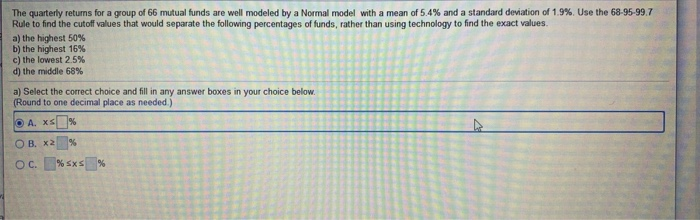The quarterly returns for a group of 66 mutual funds are well modeled by a Normal model with a mean of 5,4% and a standard deviation of 1.9%. Use the 68-95-99.7 Rule to find the cutoff values that would separate the following percentages of funds, rather than using technology to find the exact values a) the highest 50% b) the highest 16% c) the lowest 2.5% d) the middle 68% a) Select the correct choice and fill in any answer...

• ### The daily exchange rates for the​ five-year period 2003 to 2008 between currency A and currency...

The daily exchange rates for the​ five-year period 2003 to 2008 between currency A and currency B are well modeled by a normal distribution with mean 1.303 in currency A​ (to currency​ B) and standard deviation 0.036 in currency A. Given this​ model, and using the​ 68-95-99.7 rule to approximate the probabilities rather than using technology to find the values more​ precisely, complete parts​ (a) through​ (d). ​a) What would the cutoff rate be that would separate the lowest 0.15%...

• ### The closing stock price of Ahmadi, Inc. for a sample of 10 trading days is shown...

The closing stock price of Ahmadi, Inc. for a sample of 10 trading days is shown below. Day Stock Price 1 83 2 77 3 81 4 88 5 75 6 90 7 91 8 86 9 93 10 86 For the above sample, compute the following measures. a. The mean b. The median c. The mode d. The range

• ### Assume the following stock price history for the previous 10 trading days: 53.45 53.55 55.09 52.58...

Assume the following stock price history for the previous 10 trading days: 53.45 53.55 55.09 52.58 52.17 51.24 50.49 48.96 49.03 48.87 Calculate the daily holding period returns (there will be 9 of them). Then calculate the mean and standard deviation of the returns.

• ### The quarterly returns for a group of 52 mutual funds with a mean of 3.2% and...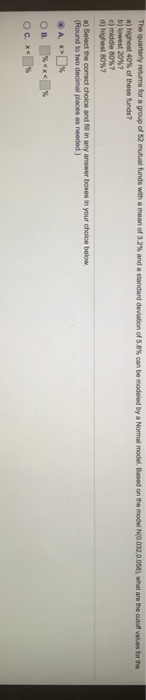The quarterly returns for a group of 52 mutual funds with a mean of 3.2% and a standard deviation of 5,8% can be modeled by a Normal model. Based on the model N(0.032,0058)what are the cutoff values for the a) highest 40% of these funds? b) lowest 20%? c) middle 80%? d) highest 80%? a) Select the correct choice and fill in any answer boxes in your choice below. (Hound to two decimal places as needed.) A > O c....

• ### Question 11 (1 point) The daily stock price for International Business Machines (IBM) historically has followed...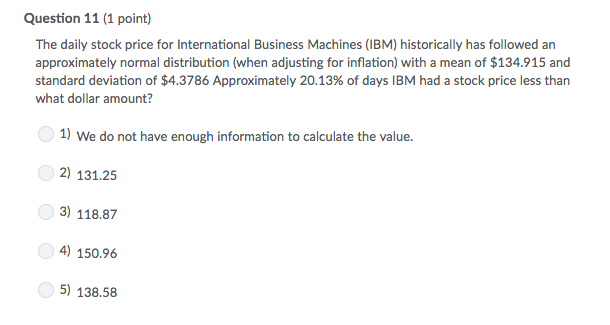Question 11 (1 point) The daily stock price for International Business Machines (IBM) historically has followed an approximately normal distribution (when adjusting for inflation) with a mean of \$134.915 and standard deviation of \$4.3786 Approximately 20.13% of days IBM had a stock price less than what dollar amount? 1) We do not have enough information to calculate the value. 2) 131.25 3) 118.87 4) 150.96 5) 138.58

• ### QUESTION 8 data The daily closing values of the Dow Jones Industrial Average over a period...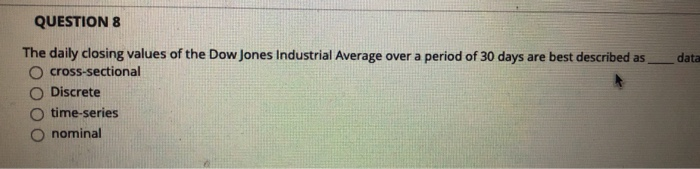QUESTION 8 data The daily closing values of the Dow Jones Industrial Average over a period of 30 days are best described as cross-sectional Discrete time-series O nominal Remaining Time: 30 minutes 5 seconds Question completion Status The daily dosing values of the Dow Jones industrial Average over a period of days we best described Cross sectional Discrete time series nominal QUESTION 9 How is the median defined if the number of observations is even? Average of the two middle...